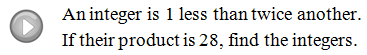Showing posts with label projectile. Show all posts
Showing posts with label projectile. Show all posts

## Monday, November 5, 2012

The applications in this chapter will involve quadratic equations. Look for the keyword “product” which indicates that we will have to multiply in our set-up. Remember to read the question several times, identify the variables and use algebra to solve the problems. Whenever possible, we will try to set them up using only one variable.

Number Problem: One positive number is 4 less than twice another positive number and their product is 96. Set-up an algebraic equation and solve it to find the two numbers.
Number Problem: The difference between two positive integers is 5 and their product is 126. Find the two integers.
Number Problem: Sarah is 2 years older than her brother Ryan. If the product of their ages is 15, how old are they?
Note: Consecutive integers are separated by 1 unit and consecutive even and odds are separated by 2 units.
Consecutive Odd Integers: The product of two consecutive odd positive integers is 99. Find the integers.
Consecutive Even Integers: The product of two consecutive even positive integers is 168. Find the integers.
Consecutive Integers: The product of two consecutive integers is 182. Find the integers.
Since this problem did not specify positive or negative integers, we must provide both sets of answers.
Basic Guidelines for Solving Word Problems:
1. Read the problem several times and organize the given information.
2. Identify the variables by assigning a letter to the unknown quantity.
3. Set up an algebraic equation.
4. Solve the equation.
5. Finally, answer the question and make sure it makes sense.

With geometry type problems it sometimes helps if we draw a picture. Here are some area formulas that you are expected to know.Geometry Problem: The length of a rectangular room is 2 feet more than twice its width. If the total area in the room is 220 square feet, find the dimensions of the room.
Geometry Problem: A square cement slab is to be surrounded by a 2-foot brick border. If the total area is to be 121 square feet, what are the dimensions of the cement slab?
Geometry Problem: A pig pen is to be built adjacent to a barn with 16 feet of fencing. If the length is to be twice that of the width, what will the area of the pen be?
Geometry Problem: The height of a triangle is 3 inches less than twice the length of its base. If the total area of the triangle is 52 square inches, find the lengths of the base and height.

When working with right triangles it is useful to remember the Pythagorean Theorem.
Geometry Problem: The hypotenuse of a right triangle is 2 centimeters more than the smallest leg. If the longer leg measures 4 centimeters, find the lengths of the other two sides.
Answer: The smaller leg measures 3 centimeters and the hypotenuse measures 5 centimeters.

Geometry Problem: The hypotenuse of a right triangle measures 10 inches. If the small leg is 2 inches less than the longer leg, find the lengths of the legs.
For the following projectile problems, use the formula for the height
where t represents time in seconds, v-naught represents the initial velocity in feet per second, and s represents the initial height in feet.

Projectile Problem: A projectile is launched from a roof 80 feet high at an initial velocity of 64 feet per second. How long will it take the projectile to land on the ground?
Projectile Problem: Old fashioned large caliber handguns (45 Colt, 44 S+W) shoot a heavy bullet without a lot of gun powder behind it. Muzzle velocities for these weapons average 1,000 feet per second. If a handgun is shot up into the air from the ground how long will it take to bullet to come back to the ground? (Wind and air resistance are not part of this calculation)
Projectile Problem: Using the previous problem, how high is the bullet at t = 30 seconds?
Projectile Problem: If a rocket is shot straight up with an initial velocity of 48 feet per second, then its height h, in feet, after t seconds is given by\
a. How high is the rocket 1/2 second after it is launched?
b. When is the rocket exactly 32 feet above the ground?
c. When will the rocket hit the ground?
Word Problem: Find a polynomial that gives the area of a circle of radius x with a square, whose diagonal measures 2x, cut from it.